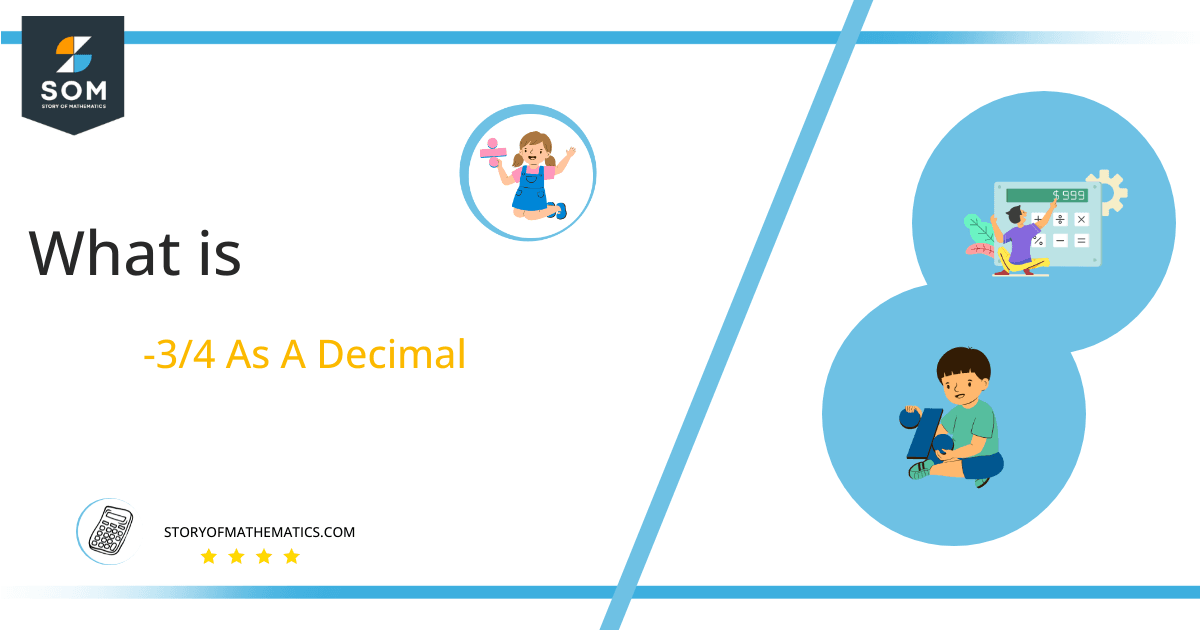# What Is -3/4 as a Decimal + Solution With Free StepsThe fraction -3/4 as a decimal is equal to 0.75.

Fractions are represented in the form of p/q where the line is the division sign that distinguishes between the numerator and denominator. The upper part of the line is known as the numerator, while the part below the line is referred to as the denominator.

Division looks a bit complex among all the mathematical operations, but actually, it is not. Because there is a way to deal with this challenging operator. We can solve fractions by using the Long Division method.

The fraction -3/4 can be converted into a decimal by using a method called the long division method.

## Solution

First, we need to understand the terms that will be used in the solution to this problem. Important terms are Dividend and Divisor. The numerator of the fraction is known as the dividend, while the denominator is referred to as the divisor. If we talk about the p/q form, then p refers to the dividend and q refers to the divisor.

Dividend = -3

Divisor = 4

Now it’s time to introduce another important term, which is the result of the problem and is known as the Quotient.

Quotient = Dividend $\div$ Divisor = -3 $\div$ 4

Now, by using the Long Division we can solve the problem as:Figure 1

## -3/4 Long Division Method

You can take a closer look at the Long Division Method utilized to fix this issue by doing the following.

-3 $\div$ 4

When we have a value of the numerator less than the denominator, we have to add the decimal point to the quotient. Here the numerator -3 is less than the denominator 4, so before starting the method we have to add the decimal point first to the quotient.

Another division-specific term, Remainder, is used, as its name shows that it is the value that remains after an incomplete division.

Here the remainder is -3, so after putting a decimal point to the quotient, we can add the zero to the remainder’s right to proceed with our solution. So now the remainder is -30.

-30 $\div$ 4 $\approx$ -7

Where:

4 x -7 = -35

This shows that a Remainder was also generated from this division, and it is equal to -2.

Here again, we have the case of a remainder less than the divisor, so we will add zero to the remainder’s right, and this time we will not add a decimal point to the quotient because it already exists there.

The remainder we get after the previous step is -2, so now by putting zero to its right, the new remainder we have is -20.

-20 $\div$ 4 = -5

Where:

4 x -5 = -20

So we have a Remainder of 0. This means this is the exact conversion of the fraction into the decimal. The resulting Quotient of the fraction by the long division method is -0.75.Images/mathematical drawings are created with GeoGebra.